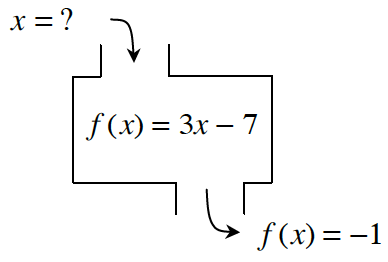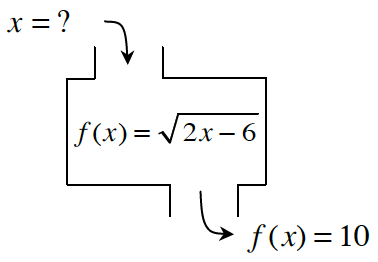### Home > CCA > Chapter 1 > Lesson 1.2.5 > Problem1-80

1-80.

Find the inputs for the following functions with the given outputs. If there is no possible input for the given output, explain why not.

1.Write out the equation.
$-1=3x-7$

Solve for $x$.
$6=3x$

$2=x$

1.Follow the steps in part (a).

$x=53$